Courses

# Algebra of Matrices - Matrices and Determinants, Business Mathematics & Statistics B Com Notes | EduRev

Created by: Arshit Thakur

## B Com : Algebra of Matrices - Matrices and Determinants, Business Mathematics & Statistics B Com Notes | EduRev

The document Algebra of Matrices - Matrices and Determinants, Business Mathematics & Statistics B Com Notes | EduRev is a part of the B Com Course Business Mathematics and Statistics.
All you need of B Com at this link: B Com
```Algebra of Matrices
```

## Addition and Subtraction of Matrices

Any two matrices can be added if they are of the same order and the resulting matrix is of the same order. If two matrices A and B are of the same order, they are said to be conformable for addition.

For example: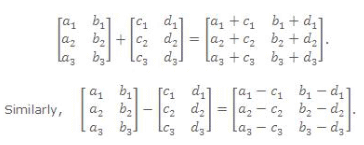```
```

### Note:

• Only matrices of the same order can be added or subtracted.

• Addition of matrices is commutative as well as associative.

• Cancellation laws hold well in case of addition.

• The equation A + X = 0 has a unique solution in the set of all m × n matrices.

### Scalar Multiplication

The matrix obtained by multiplying every element of a matrix A by a scalar λ is called the multiple of A by λ and its denoted by λ A i.e. if A = [aij] then λA = [λaij].

For example: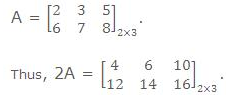```
```

Note: All the laws of ordinary algebra hold for the addition or subtraction of matrices and their multiplication by scalar.

### Multiplication of Matrices

Two matrices can be multiplied only when the number of columns in the first, called the prefactor, is equal to the number of rows in the second, called the postfactor. Such matrices are said to be conformable for multiplication.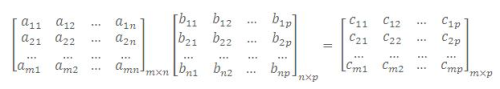where cij = ai1 b1j + ai2 b2j +...+ ain bnj= ∑nk=1  aik bki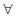i = 1, 2, 3 ......, m and
j = 1, 2, 3 ......, p.

```
```

### Properties of Multiplication

• Matrix multiplication may or may not be commutative. i.e., AB may or may not be equal to BA
• If AB = BA, then matrices A and B are called Commutative Matrices.
• If AB = BA, then matrices A and B are called Anti-Commutative Matrices.
• Matrix multiplication is Associative
• Matrix multiplication is Distributive over Matrix Addition.
• Cancellation Laws not necessary hold in case of matrix multiplication i.e., if AB = AC => B = C even if A ≠ 0.
• AB = 0 i.e., Null Matrix, does not necessarily imply that either A or B is a null matrix.

Illustration: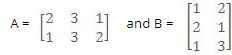show that AB ≠ BA.

Solution:

Here A.B =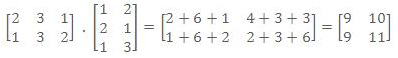and B.A =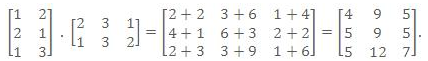```
```

Thus A.B ≠ B.A.

Illustration: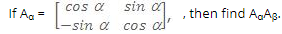Solution:

We have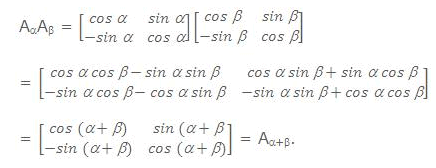Offer running on EduRev: Apply code STAYHOME200 to get INR 200 off on our premium plan EduRev Infinity!

122 videos|142 docs

,

,

,

,

,

,

,

,

,

,

,

,

,

,

,

,

,

,

,

,

,

,

,

,

;